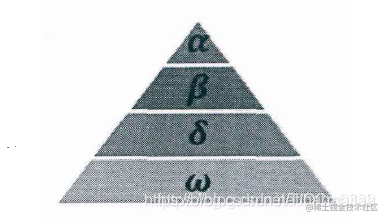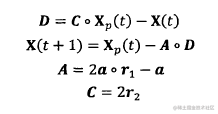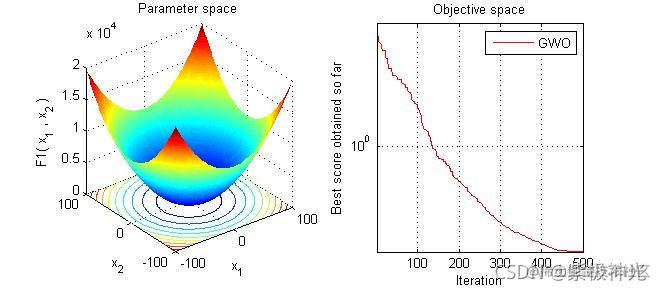# 【优化算法】灰狼优化算法（GWO）【含Matlab源码 1305期】

## 一、灰狼算法简介

1 前言： 灰狼优化算法（Grey Wolf Optimizer，GWO）由澳大利亚格里菲斯大学学者 Mirjalili 等人于2014年提出来的一种群智能优化算法。该算法受到了灰狼捕食猎物活动的启发而开发的一种优化搜索方法，它具有较强的收敛性能、参数少、易实现等特点。近年来受到了学者的广泛关注，它己被成功地应用到了车间调度、参数优化、图像分类等领域中。 2 算法原理： 灰狼隶属于群居生活的犬科动物，且处于食物链的顶层。灰狼严格遵守着一个社会支配等级关系。如图：社会等级第一层：狼群中的头狼记为 \alpha，\alpha 狼主要负责对捕食、栖息、作息时间等活动作出决策。由于其它的狼需要服从\alpha 狼的命令，所以 \alpha 狼也被称为支配狼。另外， \alpha 狼不一定是狼群中最强的狼，但就管理能力方面来说， \alpha 狼一定是最好的。

GWO 优化过程包含了灰狼的社会等级分层、跟踪、包围和攻击猎物等步骤，其步骤具体情况如下所示。

１）社会等级分层（Social Hierarchy）当设计 GWO 时，首先需构建灰狼社会等级层次模型。计算种群每个个体的适应度，将狼群中适应度最好的三匹灰狼依次标记为 \alpha、\beta 、\delta ，而剩下的灰狼标记为 \omega。也就是说，灰狼群体中的社会等级从高往低排列依次为； \alpha、\beta 、\delta 及 \omega。GWO 的优化过程主要由每代种群中的最好三个解（即 \alpha、\beta 、\delta ）来指导完成。

２）包围猎物（ Encircling Prey ）灰狼捜索猎物时会逐渐地接近猎物并包围它，该行为的数学模型如下：式中：t 为当前迭代次数：。表示 hadamard 乘积操作；A 和 C 是协同系数向量；Xp 表示猎物的位置向量； X(t) 表示当前灰狼的位置向量；在整个迭代过程中 a 由2 线性降到 0； r1 和 r2 是 [0，1] 中的随机向量。

３）狩猎（ Hunring）

４）攻击猎物（Attacking Prey）构建攻击猎物模型的过程中，根据2)中的公式，ａ值的减少会引起 A 的值也随之波动。换句话说，A 是一个在区间［-a，a］（备注：原作者的第一篇论文里这里是[-2a,2a]，后面论文里纠正为[-a,a]）上的随机向量，其中ａ在迭代过程中呈线性下降。当 A 在［-1，１］区间上时，则捜索代理（Search Agent）的下一时刻位置可以在当前灰狼与猎物之间的任何位置上。

５）寻找猎物（Search for Prey）灰狼主要依赖 \alpha、\beta 、\delta 的信息来寻找猎物。它们开始分散地去搜索猎物位置信息，然后集中起来攻击猎物。对于分散模型的建立，通过｜A｜＞１使其捜索代理远离猎物，这种搜索方式使 GWO 能进行全局搜索。GWO 算法中的另一个搜索系数是Ｃ。从2)中的公式可知，Ｃ向量是在区间范围［０，２］上的随机值构成的向量，此系数为猎物提供了随机权重，以便増加（｜Ｃ｜＞１）或减少（｜Ｃ｜＜１）。这有助于 GWO 在优化过程中展示出随机搜索行为，以避免算法陷入局部最优。值得注意的是，Ｃ并不是线性下降的，Ｃ在迭代过程中是随机值，该系数有利于算法跳出局部，特别是算法在迭代的后期显得尤为重要。

## 二、部分源代码

clear all
clc

SearchAgents_no=3; % Number of search agents

Function_name='F1'; % Name of the test function that can be from F1 to F23 (Table 1,2,3 in the paper)

Max_iteration=500; % Maximum numbef of iterations

% Load details of the selected benchmark function
[lb,ub,dim,fobj]=Get_Functions_details(Function_name);

[Best_score,Best_pos,GWO_cg_curve]=GWO(SearchAgents_no,Max_iteration,lb,ub,dim,fobj);
% Grey Wolf Optimizer
function [Alpha_score,Alpha_pos,Convergence_curve]=GWO(SearchAgents_no,Max_iter,lb,ub,dim,fobj)

% initialize alpha, beta, and delta_pos
Alpha_pos=zeros(1,dim);
Alpha_score=inf; %change this to -inf for maximization problems

Beta_pos=zeros(1,dim);
Beta_score=inf; %change this to -inf for maximization problems

Delta_pos=zeros(1,dim);
Delta_score=inf; %change this to -inf for maximization problems

%Initialize the positions of search agents
Positions=initialization(SearchAgents_no,dim,ub,lb);

Convergence_curve=zeros(1,Max_iter);

l=0;% Loop counter

% Main loop
while l<Max_iter
for i=1:size(Positions,1)

% Return back the search agents that go beyond the boundaries of the search space
Flag4ub=Positions(i,:)>ub;
Flag4lb=Positions(i,:)<lb;
Positions(i,:)=(Positions(i,:   ).*(~(Flag4ub+Flag4lb)))+ub.*Flag4ub+lb.*Flag4lb;

% Calculate objective function for each search agent
fitness=fobj(Positions(i,:));

% Update Alpha, Beta, and Delta
if fitness<Alpha_score
Alpha_score=fitness; % Update alpha
Alpha_pos=Positions(i,:);
end

if fitness>Alpha_score && fitness<Beta_score
Beta_score=fitness; % Update beta
Beta_pos=Positions(i,:);
end

if fitness>Alpha_score && fitness>Beta_score && fitness<Delta_score
Delta_score=fitness; % Update delta
Delta_pos=Positions(i,:);
end
end

a=2-l*((2)/Max_iter); % a decreases linearly fron 2 to 0

% Update the Position of search agents including omegas
for i=1:size(Positions,1)
for j=1:size(Positions,2)

r1=rand(); % r1 is a random number in [0,1]
r2=rand(); % r2 is a random number in [0,1]

A1=2*a*r1-a; % Equation (3.3)
C1=2*r2; % Equation (3.4)

D_alpha=abs(C1*Alpha_pos(j)-Positions(i,j)); % Equation (3.5)-part 1
X1=Alpha_pos(j)-A1*D_alpha; % Equation (3.6)-part 1

r1=rand();
r2=rand();

A2=2*a*r1-a; % Equation (3.3)
C2=2*r2; % Equation (3.4)

D_beta=abs(C2*Beta_pos(j)-Positions(i,j)); % Equation (3.5)-part 2
X2=Beta_pos(j)-A2*D_beta; % Equation (3.6)-part 2

r1=rand();
r2=rand();

A3=2*a*r1-a; % Equation (3.3)
C3=2*r2; % Equation (3.4)

D_delta=abs(C3*Delta_pos(j)-Positions(i,j)); % Equation (3.5)-part 3
X3=Delta_pos(j)-A3*D_delta; % Equation (3.5)-part 3

Positions(i,j)=(X1+X2+X3)/3;% Equation (3.7)

end
end
l=l+1;
Convergence_curve(l)=Alpha_score;
end

## 三、运行结果## 四、matlab版本及参考文献

1 matlab版本 2014a

2 参考文献  包子阳,余继周,杨杉.智能优化算法及其MATLAB实例（第2版）[M].电子工业出版社，2016. 张岩,吴水根.MATLAB优化算法源代码[M].清华大学出版社，2017.